## What maths should children know by the end of Year 1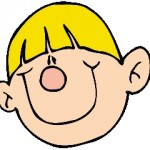To be able to successfully help your child with maths at home it is important to have a good idea of what is expected in school. Remember, we divided all the maths content into 7 strands. Let’s take each of the strands in turn to see what the Primary Framework for Mathematics suggests for children aged between 5 and 6 years old.

Counting and understanding number

By the end of year 1 children should

• know the number names (one, two, three etc) up to at least 20 and tens up to 100
(eg twenty, thirty, forty etc).

• be able to count up to 20 objects reliably. If the objects (eg buttons on a tray) are moved around they should be able to say that the total number has not changed without recounting. (Younger children will count them again if they have been moved around.)

• be able to read and write numerals up to 20
(eg 3, three, 4, four, 5 five, etc).

• be able to place numbers on a blank number line in the correct order.

• be able to say the number that is one more than or one less than any given number up to 20
(eg one less than 7).

• be able to say the number ten more than or ten less than a multiple of 10
(eg ten more than 30).

• recognise the equals sign (=) and understand it means “..is the same as..”

• use the terms, half and quarter in a practical sense
(eg give me a quarter of the orange).

Knowing and using number facts

By the end of year 1 children should

• know addition facts up to a total of 5
(eg 3 + 2).

• know all pairs of numbers with a total of 10
(eg 1 and 9, 2 and 8 etc).

• be able to work out subtraction facts from the addition facts they know.
(eg 5 -2 = 3 because 3 + 2 = 5)

• be beginning to count on and back in twos, fives and tens.

• know the doubles of all numbers up to 10
(eg double 6 is 12).

Calculating

By the end of year 1 children should

• know that addition can be done in any order.

• be able to write simple number sentences using the + and = signs
(eg 3 + 4 = 7).

• use practical methods (eg using counters) to help with addition of a 1-digit number to a 1-digit or 2-digit number
(eg 10 + 4 =).

• use informal written notes to help with addition

• understand that subtraction can mean ‘take away’.

• understand that subtraction can mean ‘find the difference between’.

• be able to use practical methods to help with subtraction of a 1-digit number from a 1-digit number
(eg using counters).

• use informal written notes to help with subtraction
(eg jotting down 5 circles and crossing out 3 for 5 – 3).

• be able to write simple number sentences using the – and = signs
(eg 7 – 4 = 3).

• understand and use the vocabulary related to addition and subtraction, including: more, add, sum, total, altogether, equals, =, take away, subtract, difference between, how much more/less, how many are left?

• be able to combine groups of 2, 5 and 10 in a practical situation
(eg combining marbles into groups of 5 marbles).

• be able to share a group of objects into 2 equal parts
(eg sweets shared equally).

Understanding shape

By the end of year 1 children should

• be able to visualise and name common 2-D shapes, including circle, triangle, rectangle and square. This includes activities such as “Find a shape which has 3 corners and 3 sides”.

• be able to visualise and name common 3-D shapes, including, cube, cuboid, sphere, cone and cylinder. This includes activities such as describing the properties of a shape by the feel of it, before seeing it.

• be able to make patterns and pictures with 2-D shapes and make models with 3-D shapes such as Lego.

• begin to describe the position of shapes, using terms such as: over, under, underneath, above, below, outside, inside, in front, behind, beside, before, after, next to, opposite, between, middle, centre, edge, corner, top, bottom, side. This is where activities such as moving furniture around a toy house are very important or placing counters on a board game.

• be using everyday language to describe direction, such as: left, right, up, down, forwards, backwards, sideways, across, along, through, to, from, towards, away from.

• begin to be able to describe movements in a straight line and turning. At first turning will only be by whole and half turns but it is important to recognise shapes that turn about a point, such as a pair of scissors.

• Once again it must be emphasised that most of this work is done orally with practical equipment. Toys, board games, may seem like playing to us but are invaluable learning experiences.

Measuring

By the end of year 1 children should
• understand and use the basic vocabulary related to length, mass and capacity.
For length: long, short, tall, high, low, wide, narrow, deep, shallow, thick, thin, far, near and words of comparison eg longer.
For mass: weight, weighs, heavy light, balances.
For capacity: full, empty, half full, holds.

• be able to make direct comparisons between two items eg comparing the length of two pencils, the weight of two parcels, the capacity of two cups. A set of balance scales is very useful for this kind of work.

• begin to use non-standard measures
(eg the jug holds 6 cups of water, the table is about 7 rulers long).

• be making sensible estimates and guesses related to length, mass and capacity. Standard units such as cm, km, kg are not used at this stage.

Using and applying maths

By the end of year 1 children should

• solve problems involving counting, addition, subtraction, doubling, halving, measures and money
(eg a toy shop, paying and giving change with numbers up to 10).

• describe and solve simple puzzles or problems
(eg How many ways can you score 4 rolling two dice?).

• sort information, shapes or objects and display the results using pictures
(eg sort cubes by colour).

• describe simple patterns involving numbers or shapes
(eg continue a pattern of repeated squares and circles).

• describe ways of solving a puzzle or problem orally
(eg talk about or draw what coins could be used to make 6p).

## Year 4 maths worksheet: decimal fractions and money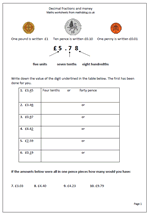Money is a great way to reinforce place value with decimals. For example in the total £3.45 the digit 4 has a value of 4 tenths or 40p. Money makes it very easy to show tenths (a 10p coin is one tenth of a pound) and hundredths (a one pence coin is one hundredth of a pound).

This page looks at this in more detail, showing that ten pence can be written in two ways; either as 10p or as part of a pound £0.10. There are two further things to remember here:

1. that we should always have two digits after the decimal point when writing fractions of a pound eg £0.30 not £0.3.

2. that we should not include both the pound and pence sign when writing amounts in pounds eg £0.30 is correct but £0.30p is not. it is 0.30 of a pound, not 0.30 of a penny.

This is just one worksheet from a great selection in our Counting and Understanding Numbers section within our year 4 maths worksheets. Why not go and have a look at what else is freely available?

Decimal fractions and money

## Year 2 maths worksheets: symmetry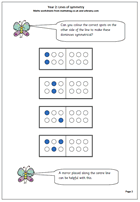Folding to create symmetrical patterns is a popular past-time in schools. These maths worksheets for year 2 are quite tricky as they ask children to colour the correct spots on the other side of the line of symmetry to make sure that the shape is symmetrical.

Pegboards are a useful aid to this work, as is a mirror, which can be laid along the line, or axis of symmetry to see how the pattern continues.

More can be found in our year 2, Understanding shape section.

symmetry_1

## Year 4 Handling Data: information from a table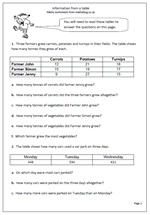As adults we read and interpret information from tables and charts almost on a daily basis, but children need practice at this. To answer the questions on this worksheet children have to understand the layout of the table and interpret the figures. It also needs a nifty bit of adding three numbers, so jottings might well be the order of the day.

This worksheet can be found in our Year 4 Handling Data category.

Information from a table

## Counting in halves and quarters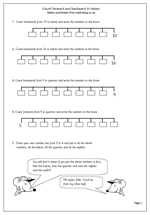Here is a worksheet that is difficult to find anywhere else, but covers an important aspect of counting ie counting in halves and quarters. The sheet looks at counting forwards and backwards and certainly the counting back in quarters requires concentration.

The final question asks for a number line from 0 to 4 to be drawn and then all the whole numbers, halves, quarters and intermediate eighths to be included. Completing this successfully will show a good understanding of quarters and eighths ie that 1/2 is the same as 4/8 etc.

This can be found in our year 3, Counting and Number category

Count forward and backward in halves and quarters

## What maths should children know by the end of reception?Let’s begin by looking at what is expected by the end of the Reception year – that’s children aged between 4 and 5 years old. This is sometimes called the Foundation Stage. Yes, even our 5 year olds have targets to reach!
I will take each of the strands in turn, but as you go through them it will become clear that the most important thing of all is to talk with your children and use the vocabulary of simple maths to help them describe the world around them.

Counting and understanding number:

• Children should be able to say and use number names up to 10. A good example of this would be to join in nursery rhymes or songs such as:
“One, two three, four, five. Once I caught a fish alive.”

• Children should be beginning to count up in ones, up to 10 objects. This would be in a practical sense of counting a number of objects that they can touch (pieces of a jigsaw, coins, hats etc). Later they can count things they can see, but not touch (panes in a window, cows in a field etc).They can then begin to count down from a small number eg 5,4,3,2,1,0.

• They should be beginning to use mathematical language to compare two numbers. Words to include are: bigger/smaller, more/less, first/last.

• Recognise the numerals 1 to 9. Birthday cards with the age on is always a good starting point. Make a set of numbers from 0 to 9 on card. Pick out the numbers in a story: 3 Billie Goats Gruff etc.

Knowing and using number facts:

• Children should know one more or one less than a number between 1 and 10. In other words they know that 5 is one more than 4 etc. Again, the real world is the best learning environment – laying the table, in the kitchen etc.

Calculating:

• Children should begin to see addition as the combining of two groups of objects. (An example of this might be “Show me 3 fingers on your right hand. Show me two fingers on your left hand. How many fingers are showing altogether? Count 123….12….12345. They will need the oral prompt of “so 3 fingers and 2 fingers make 5 fingers” after the counting.)

• Children should begin to see subtraction as ‘taking away’. (When asked how many are left after eating 2 of our 5 cakes to begin with children will do this by counting how many are left. Having counted what is left, they need the reinforcement orally -“let’s say together 5 take away 2 is 3”.

• Through practical activities they will begin to use the vocabulary of addition and subtraction: add, make, more, take away, how many are left?

• A further extension is to begin to share objects into equal groups and count how many there are in each group.

Understanding shape:

• Children are expected to be able to create simple patterns. Much of this might be described as art work – using sets of shapes, printing, beads, cotton reels etc to create simple patterns and talk about them.

• Children are expected to be able to use the vocabulary of shape to describe the shape and size of solid and flat shapes: circle, triangle, square, rectangle, cube, cone and sphere. These could be shapes they make themselves or familiar shapes found around the home: mats, boxes etc.

• Use everyday words to describe the position of objects. A wide vocabulary including: over, under, above, below, next to, in front, behind, outside, inside, next to, left, right, up, down, forwards, backwards, across. Reading books are excellent for this type of work: looking at a picture in a book such as “Where’s Spot”.

Measuring:

• Children are expected to use the vocabulary of simple measurement, such as: more or less, longer or shorter, greater or smaller, heavier or lighter, thick, thin, tall, high, low, full, empty. Much of this is in the form of comparisons and needs to be done on a practical basis: a pair of balance scales and empty containers which can be filled with sand/water are really useful for this.

• Children are expected to use the vocabulary of time. They are expected to begin to know the days of the week, morning, afternoon, night, today, tomorrow, yesterday, now, soon, later, before, after, next, last. Again, story books are a superb resource such as The Very Hungry Caterpillar by E Carle. They should be beginning to use hours of the day: my bedtime is 8 o’clock etc.

Handling data:

• Children are expected to be able to sort familiar objects according to simple criteria – colour, shape, size etc. A tray of beads, lego, coins, marbles etc are all useful for this.
• They will count the number of objects which share a particular property (eg red crayons)
• They will also begin to present results of their counting using pictures or drawings

Using and applying mathematics:

• Use mathematical ideas to solve simple practical problems
• Match sets of objects to numerals
• Sort objects
• Talk about patterns in number
• Talk about how to solve a simple practical problem.

## Mr Magnolia by Quentin Blake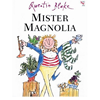Mister MagnoliaWritten in the 1980s this is a great little story that is excellent for encouraging understanding of pairs and odd and even numbers. In this book Mr Magnolia leads a really happy life apart from one rather strange thing – he only has one boot. Written more or less as nonsense verse it appeals to children with imagination as he has a number of very strange possessions, including a frog, a toad and a newt, some exotic birds a big purple dinosaur who’s a magnificent brute.

Quentin Blake is, of course, one of our best known illustrators, having illustrated over 300 books, including many of Roald Dahl’s.

## KS2 maths test questionsDon’t forget that we have some excellent ‘booster’ pages for children who will be doing their SATs this summer. Here we have some examples of the type of arithmetic questions that come up. An interesting example is the question which asks to put the digits 7, 3 and 4 into the boxes to make the total of 41.

Sometimes there is more than one way to do this type of question and it is a matter of using ‘trial and improvement’ to solve it.

One good way to tackle it is to look at the units (in this case 1) and see which two digits added together will put a 1 in the units. The only option here is is to add the digits 7 and 4 (which make 11), put these in the units boxes and it is easy from there on.

Thanks to MathSphere for letting us use this worksheet.

Booster maths worksheet 3

## Year 5 ordering fractions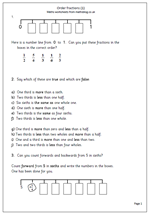Fractions always seem to cause problems for children who are less confident with their maths, often because they do not get enough practice with ordering and comparing fractions. This worksheet compares sixths and thirds by placing them in order on a number line as well as asking whether statements are true or false. A good understanding of equivalent fractions is needed to do this and many children will still need practice at counting forwards and backwards in fractions (eg count on from zero in sixths up to 2 whole ones.)

Order fractions (1)

## Year 4 maths worksheet: easy or not?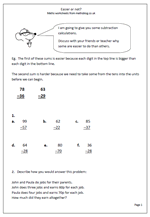Here is a very different page of maths. We all  know that some subtractions are harder than others, even if the numbers are of a similar size, but why? Our worksheet asks children this very question, and hopefully they will be able to give some sensible reasons.

When it comes to written subtraction some children will always write down the difference between the two digits on the top and bottom lines. Sometimes this is correct (if the digit on the top line is bigger), but often it is not correct (if the digit on the bottom line is bigger) as ‘decomposition’ is needed to work out the answer. This page should show whether children are aware of this.

The second part of the page asks children how they would set about tackling a question; note this does not mean that the correct answer has to be worked out, just a correct method. It will show a great deal about children’s thinking and confidence.

This can be found in our Using and Applying Maths section of Year 4 maths worksheets.

Easier or not